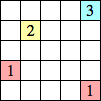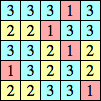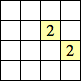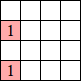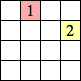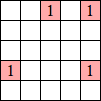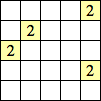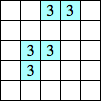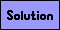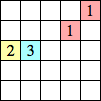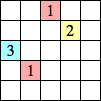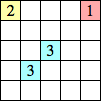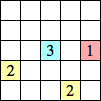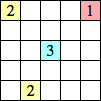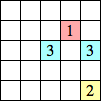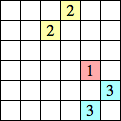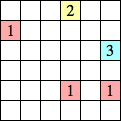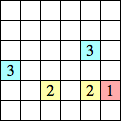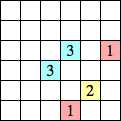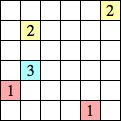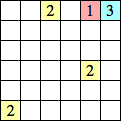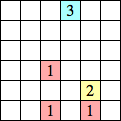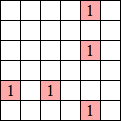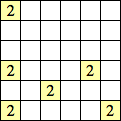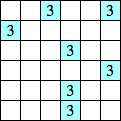123 Puzzles

Put a 1, 2, or 3 in every blank square. Each number indicates how many squares are part of the horizontally and vertically adjacent group with the same number. That is, groups of 1's, 2's and 3's contain exactly 1, 2, and 3 squares respectively. A sample puzzle is shown below. Each puzzle below has a unique solution.